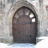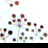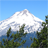# Distance between related points

2651
15
12-05-2012 07:55 AMNew Contributor
Here is the thing, I have two point shapes, one containing ???proposed??? sampling stations and the second one with the ???real??? coordinates where the samples were taken. The proposed and real points are linked together by a common code (5K_ID). What I need is to calculate the distance from all points in the ???proposed??? dataset to the corresponding point (by 5K_ID) in the ???real??? dataset. Any help would be much appreciated ???
Tags (3)
15 RepliesRegular Contributor II
use GenerateNearTable using the same FC for both in_features and near_features and the 'ALL' option on closest.
That give you a (potentialy very big) table of distances between every point and every other.
You can also get the bearing angle too, if you want.

PointDistance may work for you too.

You may want to make selections on the 5K_ID field before running it, to get (many) smaller tables to work with.

Without that:

AddXY_management will give you locations for each point.
You could then do some trig on the X,Y location differences between pairs of matched pointsNew Contributor
use GenerateNearTable using the same FC for both in_features and near_features and the 'ALL' option on closest.
That give you a (potentialy very big) table of distances between every point and every other.
You can also get the bearing angle too, if you want.

PointDistance may work for you too.

You may want to make selections on the 5K_ID field before running it, to get (many) smaller tables to work with.

Without that:

AddXY_management will give you locations for each point.
You could then do some trig on the X,Y location differences between pairs of matched points

Thx for the answer, I have already done what you suggested, but as you pointed out I get a huge table containing distances to all the possible combinations and the process takes a lot to execute, Now I need either a way to calculate distances only to the corresponding points or a way to filter the result table to get only those results I need.byEsri Regular Contributor
Here is a workaround idea:

1. Make sure the two sets of points are in the same projection/coordinate system; then use the Merge tool to combine them into one input.
2. Use the Points To Line tool with the 5k_ID as the Line Field to obtain lines between each pair of proposed and real points. The line output carries the 5k_ID field and the Shape_Length values would be the distances you need. For shapefile output, you would need to add a field and then calculate the shape length, using the Add Field and Calculate Field tools.
3. Run the Join Field tool to join the line output with any of the two point inputs via the common 5K_ID to transfer the Shape_Length field to it.

Does it work for you?byMVP Honored Contributor
Along the lines of Dan's idea, you could Add XY Coordinates to each of your point feature classes, Join one to the other by the common field, then use Geometry to calculate the distance between the two sets of coordinates. As long as your feature classes are in the same projection, I'm pretty sure this is valid (although I'm open to explanations as to why not), but you should double-check a few pairs in ArcMap with the distance tool.New Contributor
Here is a workaround idea:

1. Make sure the two sets of points are in the same projection/coordinate system; then use the Merge tool to combine them into one input.
2. Use the Points To Line tool with the 5k_ID as the Line Field to obtain lines between each pair of proposed and real points. The line output carries the 5k_ID field and the Shape_Length values would be the distances you need. For shapefile output, you would need to add a field and then calculate the shape length, using the Add Field and Calculate Field tools.
3. Run the Join Field tool to join the line output with any of the two point inputs via the common 5K_ID to transfer the Shape_Length field to it.

Does it work for you?

I´m so far sounds like a good way to get it... i{ll let you know if have any more problems.. THX for the helpFrequent Contributor II
Samuel,

Have you had a look at Hawths analysis tools - Distance between Points tool:

http://www.spatialecology.com/htools/pntdistbetw.php

Regards

AnthonybyEsri Regular Contributor
If Anthony's or Dan's suggestion hasn't solved your issue - please let me know which version of ArcGIS you are using.New Contributor
Samuel,

Have you had a look at Hawths analysis tools - Distance between Points tool:

http://www.spatialecology.com/htools/pntdistbetw.php

Regards

Anthony

In fact I have Anthony but the problem is the same than with the point distance tool... The output tells me the distance to any other point in the shape and I need to know only the distance to one of them. Having more than 5K pints on my shapes makes unpractical to do it in that way.

As for the "point to line" method, it does solve the problem to some extent but it has one important limitation and that is that the two 5K codes used to generate the lines must be identical and here is where my problem gets more complicated...

Let´s add another variable to the original problem. In the original shapes, the two related points were named the same (same 5k_ID) and I was looking for a way to calculate distance from point "a" in shape "A" to shape "a" on shape "B", but now I have another field on my shape (or another table/shape) where the points are related in such a way that the 5_KID´s don match any more. And I must calculate the distance from point �??a�?� on shape �??A�?� to the point �??b�?� on shape �??B�?� and I need to do it once a day for 5000 points...

NobbirAhmed

If Anthony's or Dan's suggestion hasn't solved your issue - please let me know which version of ArcGIS you are using.

I'm on ArcGis 10.1 and have Advanced Lic.

BTW:
Coordinated system and datum�??s are no problembyEsri Regular Contributor
Are the 5k_ID values unique for each of the point feature classes?

If possible, could you please share your data (keeping only the 5k_ID field)? You can send the data to my email as well: nahmed@esri.com

It will be easier if you use Python script - do you want that?